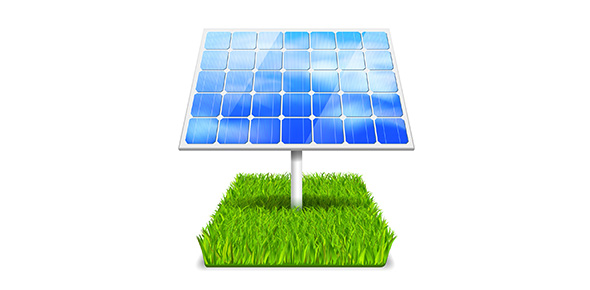# What Is Energy?

9 Questions | Total Attempts: 38SettingsThe quiz is about what energy is

Related Topics
• 1.
What is energy?
• A.

Stuff they put in drinks to make you happy

• B.

The abillity to cause change

• 2.
Kinetic energy INCREASES as...
• A.

Weight increases

• B.

As height increases

• C.

As mass increases

• D.

As speed increases

• 3.
As thermal energy increases
• A.

Heat increases

• B.

Speed increases

• C.

Mass increases

• 4.
Chemical energy is an example of
• A.

Food

• B.

A book on a shelf

• C.

A rock on a cliff

• 5.
A battery is an example  of what energy?
• A.

Chemical

• B.

Thermal

• C.

• 6.
A bowling ball moving is...
• A.

Kinetic

• B.

Potential

• C.

Thermal

• 7.
A book on a shelf is what energy
• A.

Potential

• B.

Thermal

• C.

Kinetic

• 8.
At the top of a roller coaster is the greatest ------ energy
• A.

Kinetic

• B.

Thermal

• C.

Potential

• 9.
Pizza is -------- energy
• A.

Thermal

• B.

Chemical

• C.

Potential